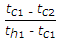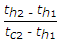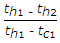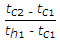# Mechanical Engineering - Heat Transfer, Refrigeration and Air Conditioning

### Exercise :: Heat Transfer, Refrigeration and Air Conditioning - Section 7

11.

During sensible cooling of air, specific humidity

 A. remains constant B. increases C. decreases

Explanation:

No answer description available for this question. Let us discuss.

12.

The transfer of heat by molecular collision is known as

 A. conduction B. convection C. radiation D. none of these

Explanation:

No answer description available for this question. Let us discuss.

13.

When tc1 and tc2 are the temperatures of cold fluid at entry and exit respectively and th1 and th2 are the temperatures of hot fluid at entry and exit point, and cold fluid has lower heat capacity rate as compared to hot fluid, then effectiveness of the heat exchanger is given by

 A.B.C.D.Explanation:

No answer description available for this question. Let us discuss.

14.

In a psychrometric chart, specific humidity (moisture content) lines are

 A. vertical and uniformly spaced B. horizontal and uniformly spaced C. horizontal and non-uniformly spaced D. curved lines

Explanation:

No answer description available for this question. Let us discuss.

15.

The expression Q = ρ AT4 is called

 A. Fourier equation B. Stefan-Boltzmann equation C. Newton-Rikhmann equation D. Joseph-Stefan equation